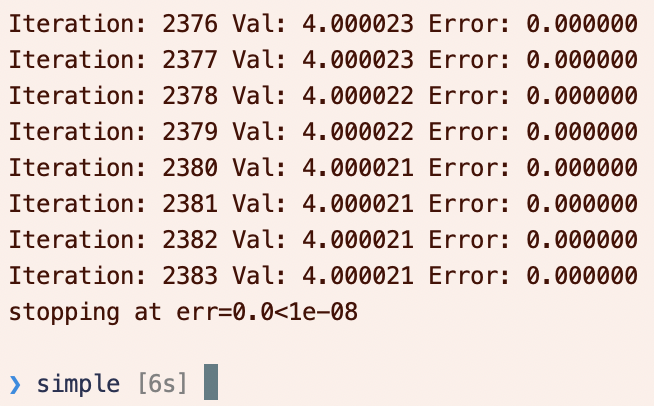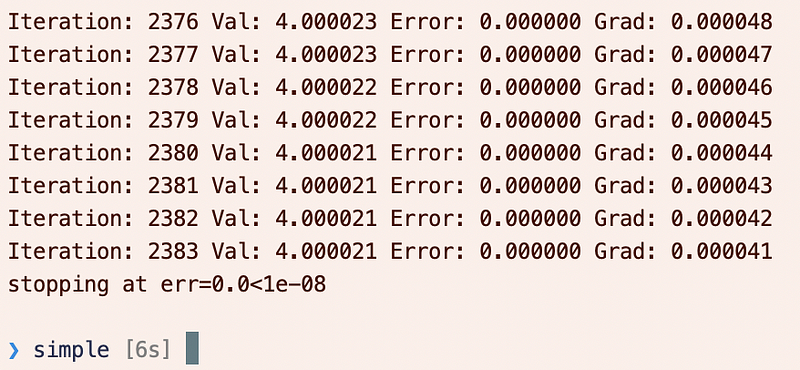A simple optimization example with Tensorflow 2.0.I’ve often used tf.GradientTape from StackOverflow snippets, hastily glued together. I’ve also often used tf.GradientTape , and the minimize method usually appears. Finally I’ve separated the two in my mind in a simple application: optimizing a 1D function, e.g. (x-4)**2 in one scalar variable x.

The relationship between the two approaches is actually quite simple:

• One optimization method is to use the minimize method, as documented here, which performs two steps:
1. It uses tf.GradientTape to calculate the gradient.
2. It uses apply_gradients to apply the gradients to the parameters.
• The other method is to unpack the action of the minimize method and manually perform these two steps.

# Optimization using minimize

As a simple example, consider minimizing (x-4)**2 in one scalar variable x . To do so, we define a loss_function() , which must take no arguments.

• loss_function is the loss function
• run is the run function starting from an initial guess.
• Iteratively, we apply the opt.minimize(loss_function, var_list=[x]) function to minimize the loss function with respect to the variable list.
• We stop if the error tolerance is sufficiently low.
• minimize automatically updates the iterations (actually: apply_gradients is updating the iterations, which is called by minimize ), and we stop if we hit the limit.

The output should be something like the following:Alternatively, we can use the tf.GradientTape and apply_gradients methods explicitly in place of the minimize method.

• We no longer need a loss function.
• We use with tf.GradientTape(persistent=False) as t to create the tape, and then t.gradient(y,[x]) to calculate the gradient in y with respect to x .
• Note: you may be surprised that we can exit the with indentation and still access the tape t ! In this case, you need to familiarize yourself with “blocks” as discussed here. The scope of variables extends throughout a block, which is either a module, function or a class. Since the with statement is not one of these, then you can still access the gradient tape! You may have previously seen the with statement used like this:
with open(fname,"r") as f:
...


But even here, even though the file is closed when the with statement ends, the variable f is not out of scope.

• We apply the gradients to x with opt.apply_gradients(zip(gradients,[x])) .

The output should be similar, but now we also have gradient information:# Final thoughts

Pretty simple, really!

A couple last notes on tf.GradientTape :

• Most times you do not need to call t.watch(x) — from the documentation, “By default GradientTape will automatically watch any trainable variables that are accessed inside the context.” You can disable this in the constructor of the tf.GradientTape .
• When do you need to use persistent=True : you really only need this when you have defined an intermediate variable that you also want the gradient with respect to. For example, the following will **not** work:
with tf.GradientTape(persistent=False) as t:
z = x-4
y = z**2


Even though t is still in scope after the with block (see discussion above), from the documentation: “By default, the resources held by a GradientTape are released as soon as GradientTape.gradient() method is called.”. So the second gradient call will fail.

with tf.GradientTape(persistent=True) as t:
z = x-4
y = z**2

# Don't forget now to do garbage collection when done computing gradients!

Don’t forget the del t after you’ve computed all the gradients you’re interested in.